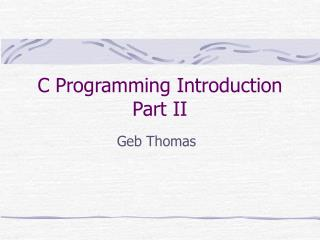DownloadDownload PresentationC Programming Introduction Part II

# C Programming Introduction Part II

Download Presentation## C Programming Introduction Part II

- - - - - - - - - - - - - - - - - - - - - - - - - - - E N D - - - - - - - - - - - - - - - - - - - - - - - - - - -
##### Presentation Transcript

1. C Programming IntroductionPart II Geb Thomas

2. Learning Objectives • Learn how to use C functions • Learn how to use C arrays • Understand what a pointer is and how to use it • Understand how to open a file and write to it.

3. C Functions • Generally, you pass variables to a function, it does some work, and returns a variable. • double Sqrt(double val) – a function that takes a value and returns its square root. • double pow(double v1, double v2) – a function that raises v1 to the power v2. • Sometimes you don’t need or want a return value (such as the function to close a file). • Sometimes you don’t need to pass anything to the function or get anything back (such as a function to clear the monitor of any data).

4. Declaring C Functions • All functions should be declared at the top of the program (before they are used). • int count(void); • int openFile(char *fileName); • The declaration tells the compiler how the function should be used -- what it should take and what it should return.

5. Defining Functions • The function is defined in the body of the c-file, outside of any other functions. • The definition explains what the function does with the information passed in and how it determines the information to return. int factorial(int inVal) { /* start of function definition */ int cntr, returnVal = 1; /*declaration of local variables */ for (cntr = 2; cntr <= inVal; cntr++) /* note use of “inVal” */ returnVal = returnVal*cntr; return returnVal; /* function will return this value */ } /* end of function definition */

6. Using Functions • To use a function, just pass variables or arguments in the correct order. • You can use the return value to set another variable. int main(int argc, void **argv) { int a = 4, b; b = factorial(a); b = factorial(6); }

7. C Arrays • C uses square brackets for defining and using arrays. • double F; declares a six-dimensional vector. • The six values in f are ordered starting from 0. • F = 3; /*set 1st element to 3. */ • F = 14.1234; /* set 4th element to 14 … */ • F = 234.341; /* error, this would be the 7th element in a six-dimensional vector */ • You can pass element values to functions • F = sqrt (F);

8. Pointers • C allows the programmer to access specific locations in memory with pointers. • Pointers user two operators: • & the “address of” operator • * the “indirection” or “dereferencing” operator

9. How Pointers Work • Instead of referring to a variable directly, pointers provide the location where the variable exists in memory. • This is useful when you want to change a variable in memory within a function. • Normally a function copies its arguments and changes to the passed arguments to not affect the main program. • If you pass a pointer, then when you change the values at the address pointed to, the changes DO affect the main program.

10. How to Use Them • Declare a pointer to a float: • float *floatPtr; • Declare a pointer to a double; • double *dblPtr; • Set the pointer to a variable. • floatPtr = &myFloatVariable; • Set the value in the pointer • *floatPtr = 6; • Use the value in a pointer • printf(“Pointer value is %f\n”, *floatPtr);

11. File Access • FILE *fptr; /* pointer to a file structure */ • fptr = fopen(“filename”, “r+”); • fprintf(“Print this %f, baby\n”, val); • fscanf(“%f”, &val); /* reads a value */ • fclose(fptr);

12. Learning Objectives • Learn how to use C functions • Learn how to use C arrays • Understand what a pointer is and how to use it • Understand how to open a file and write to it.